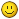# Z Touch Off

• cmorley
• Offline
• Moderator
•01 Sep 2022 03:59 #250908 by cmorley
Replied by cmorley on topic Z Touch Off
Other file
##### Attachments:

• cmorley
• Offline
• Moderator
•01 Sep 2022 04:00 #250909 by cmorley
Replied by cmorley on topic Z Touch Off
the files names are to be:
probe_z.ui
probe_z_handler.py

01 Sep 2022 16:58 #250935 by dzawacki
Replied by dzawacki on topic Z Touch Off
Thanks Chris for putting this together, I appreciate your help so far.

I've installed the two files and updated the .ini file as instructed. When running LinuxCNC from the terminal, I get the following errors:
```/usr/bin/gladevcp:221 RuntimeWarning: missing handler 'on_button_probe_z_clicked'
builder.connect_signals(handlers)
HAL: ERROR: duplicate component name 'gladevcp'

I doublechecked the probe_z_handler.py and the function for the handler is defined and using the same name. Nothing else seems off, so I'm not sure if something jumps out to you?

• cmorley
• Offline
• Moderator
•02 Sep 2022 02:07 #250967 by cmorley
Replied by cmorley on topic Z Touch Off
could you post the modified INI you used?

02 Sep 2022 13:48 #251004 by dzawacki
Replied by dzawacki on topic Z Touch Off
Sure, here it is:
```# Generated by PNCconf at Mon Aug 29 11:34:47 2022
# Using LinuxCNC version:  2.8
# If you make changes to this file, they will be
# overwritten when you run PNCconf again

[EMC]
MACHINE = WoodSmith_2x4_CNC
DEBUG = 0
VERSION = 1.1

[DISPLAY]
DISPLAY = axis
POSITION_OFFSET = RELATIVE
POSITION_FEEDBACK = ACTUAL
MAX_FEED_OVERRIDE = 2.000000
MAX_SPINDLE_OVERRIDE = 1.000000
MIN_SPINDLE_OVERRIDE = 0.500000
INTRO_GRAPHIC = linuxcnc.gif
INTRO_TIME = 5
PROGRAM_PREFIX = /home/danny/linuxcnc/nc_files
INCREMENTS = 1in .25in .1in .05in .01in .005in .001in .0005in .0001in
POSITION_FEEDBACK = ACTUAL
DEFAULT_LINEAR_VELOCITY = 1.250000
MAX_LINEAR_VELOCITY = 2.500000
MIN_LINEAR_VELOCITY = 0.016670
DEFAULT_ANGULAR_VELOCITY = 12.000000
MAX_ANGULAR_VELOCITY = 180.000000
MIN_ANGULAR_VELOCITY = 1.666667
EDITOR = gedit
GEOMETRY = xyz

[FILTER]
PROGRAM_EXTENSION = .png,.gif,.jpg Greyscale Depth Image
PROGRAM_EXTENSION = .py Python Script
png = image-to-gcode
gif = image-to-gcode
jpg = image-to-gcode
py = python

CYCLE_TIME = 0.010

[RS274NGC]
PARAMETER_FILE = linuxcnc.var
RS274NGC_STARTUP_CODE = G20 G40 G90 G94 G97 G64 P0.001

[EMCMOT]
EMCMOT = motmod
COMM_TIMEOUT = 1.0
SERVO_PERIOD = 1000000

[HMOT]
# **** This is for info only ****
CARD0=hm2_7i96.0

CARD0=hm2_7i96.0

[HAL]
HALUI = halui
HALFILE = WoodSmith_2x4_CNC.hal
HALFILE = custom.hal
POSTGUI_HALFILE = postgui_call_list.hal
SHUTDOWN = shutdown.hal

[HALUI]
MDI_COMMAND = G10 L20 P0 Z0 ( Set Z to zero )

[KINS]
JOINTS = 3
KINEMATICS = trivkins coordinates=XYZ

[TRAJ]
COORDINATES =  XYZ
LINEAR_UNITS = inch
ANGULAR_UNITS = degree
DEFAULT_LINEAR_VELOCITY = 0.20
MAX_LINEAR_VELOCITY = 2.00
POSITION_FILE = position.txt

[EMCIO]
EMCIO = io
CYCLE_TIME = 0.100
TOOL_TABLE = tool.tbl

#******************************************
[AXIS_X]
MAX_VELOCITY = 2.0
MAX_ACCELERATION = 50.0
MIN_LIMIT = -0.0
MAX_LIMIT = 43.5

[JOINT_0]
TYPE = LINEAR
HOME = 1.0
FERROR = 0.5
MIN_FERROR = 0.05
MAX_VELOCITY = 2.0
MAX_ACCELERATION = 50.0
# The values below should be 25% larger than MAX_VELOCITY and MAX_ACCELERATION
# If using BACKLASH compensation STEPGEN_MAXACCEL should be 100% larger.
STEPGEN_MAXVEL = 2.50
STEPGEN_MAXACCEL = 62.50
P = 1000.0
I = 0.0
D = 0.0
FF0 = 0.0
FF1 = 1.0
FF2 = 0.0
BIAS = 0.0
MAX_OUTPUT = 0.0
# these are in nanoseconds
DIRSETUP   = 5000
DIRHOLD    = 5000
STEPLEN    = 5000
STEPSPACE  = 5000
STEP_SCALE = 4063.9999
MIN_LIMIT = -0.0
MAX_LIMIT = 43.5
HOME_OFFSET = 0.000000
HOME_SEARCH_VEL = -1.250000
HOME_LATCH_VEL = 0.316667
HOME_FINAL_VEL = 0.750000
HOME_USE_INDEX = NO
HOME_IGNORE_LIMITS = YES
HOME_SEQUENCE = 1
#******************************************

#******************************************
[AXIS_Y]
MAX_VELOCITY = 2.0
MAX_ACCELERATION = 50.0
MIN_LIMIT = -0.0
MAX_LIMIT = 23.75

[JOINT_1]
TYPE = LINEAR
HOME = 1.0
FERROR = 0.5
MIN_FERROR = 0.05
MAX_VELOCITY = 2.0
MAX_ACCELERATION = 50.0
# The values below should be 25% larger than MAX_VELOCITY and MAX_ACCELERATION
# If using BACKLASH compensation STEPGEN_MAXACCEL should be 100% larger.
STEPGEN_MAXVEL = 2.50
STEPGEN_MAXACCEL = 62.50
P = 1000.0
I = 0.0
D = 0.0
FF0 = 0.0
FF1 = 1.0
FF2 = 0.0
BIAS = 0.0
MAX_OUTPUT = 0.0
# these are in nanoseconds
DIRSETUP   = 5000
DIRHOLD    = 5000
STEPLEN    = 5000
STEPSPACE  = 5000
STEP_SCALE = 4063.9999
MIN_LIMIT = -0.0
MAX_LIMIT = 23.75
HOME_OFFSET = 0.000000
HOME_SEARCH_VEL = -1.250000
HOME_LATCH_VEL = 0.316667
HOME_FINAL_VEL = 0.750000
HOME_USE_INDEX = NO
HOME_IGNORE_LIMITS = YES
HOME_SEQUENCE = 2
#******************************************

#******************************************
[AXIS_Z]
MAX_VELOCITY = 0.833333333333
MAX_ACCELERATION = 25.0
MIN_LIMIT = -6.0
MAX_LIMIT = 0.0

[JOINT_2]
TYPE = LINEAR
HOME = -1.0
FERROR = 0.5
MIN_FERROR = 0.05
MAX_VELOCITY = 0.833333333333
MAX_ACCELERATION = 25.0
# The values below should be 25% larger than MAX_VELOCITY and MAX_ACCELERATION
# If using BACKLASH compensation STEPGEN_MAXACCEL should be 100% larger.
STEPGEN_MAXVEL = 1.04
STEPGEN_MAXACCEL = 31.25
P = 1000.0
I = 0.0
D = 0.0
FF0 = 0.0
FF1 = 1.0
FF2 = 0.0
BIAS = 0.0
MAX_OUTPUT = 0.0
# these are in nanoseconds
DIRSETUP   = 5000
DIRHOLD    = 5000
STEPLEN    = 5000
STEPSPACE  = 5000
STEP_SCALE = -4063.9999
MIN_LIMIT = -6.0
MAX_LIMIT = 0.0
HOME_OFFSET = 0.000000
HOME_SEARCH_VEL = 0.816667
HOME_LATCH_VEL = 0.316667
HOME_FINAL_VEL = 0.500000
HOME_USE_INDEX = NO
HOME_IGNORE_LIMITS = YES
HOME_SEQUENCE = 0
#******************************************

[SPINDLE_0]
P = 0.0
I = 0.0
D = 0.0
FF0 = 1.0
FF1 = 0.0
FF2 = 0.0
BIAS = 0.0
MAX_OUTPUT = 2000.0```

I'm only seeing it referenced in the DISPLAY area, and there's a lot going on in the EMBED_TAB_COMMAND to the point that it looks like too many to me, but I have no idea.

• cmorley
• Offline
• Moderator
•02 Sep 2022 16:32 #251021 by cmorley
Replied by cmorley on topic Z Touch Off
OK comment out:
EMBED_TAB_NAME
EMBED_TAB_COMMAND

We will concentrate on the line:

Sorry for the back and forth - always harder to trouble shoot across the internet06 Sep 2022 22:37 #251361 by andypugh
Replied by andypugh on topic Z Touch Off
Have you tried the sample config (for Axis)

sim/axis/remap/manual-toolchange-with-tool-length-switch/